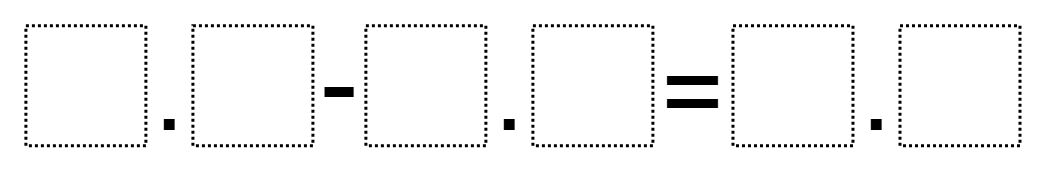Home > Grade 5 > Subtracting Decimals To Get Close To 0

# Subtracting Decimals To Get Close To 0

Directions: Using the digits 1-9, subtract two numbers to get a difference closest to 0.### Hint

What is your plan for the digits? Where should you place them? What does it mean for a decimal to be close to 0?

There could be multiple answers, but we have only found one so far. It is 21 – 19.8 = 1.2

Source: Owen Kaplinsky

## Balanced Equations 2

Directions: Use the operation symbols (+, -, x, ÷) and equal sign (=) to make …

1.Why is the difference (answer) shifted to the right so that the decimal points are misaligned? Are the instructions to use digits more than once? Or can you use the digits only one time? The answer shows multiple up to 3 digits being used, yet the example leads people to use only two digit numbers at each level. Finally, the answer shows 21 as the minuend, yet the example falsely makes me think that I should be using a decimal, like 2.1. Thank you.

•Robert Kaplinsky

Sorry, we’ve fixed this image.

2.•Robert Kaplinsky

Sorry, we’ve fixed this image.

•Sorry…. I don’t see how this image was corrected. It looks like the same problem.

3.4. 12 – 3.98 is what I found.

•Nice! That’s what I got too!

4.Nice! That’s what I got too!